Скачать презентацию Chapter 4 UTILITY MAXIMIZATION AND CHOICE MICROECONOMIC THEORY

a441f654cda9c3bc5637df894a33de59.ppt

• Количество слайдов: 40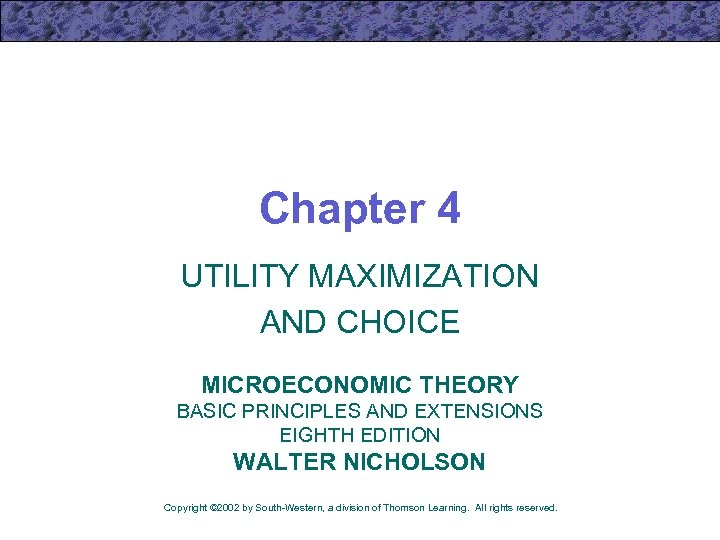Chapter 4 UTILITY MAXIMIZATION AND CHOICE MICROECONOMIC THEORY BASIC PRINCIPLES AND EXTENSIONS EIGHTH EDITION WALTER NICHOLSON Copyright © 2002 by South-Western, a division of Thomson Learning. All rights reserved.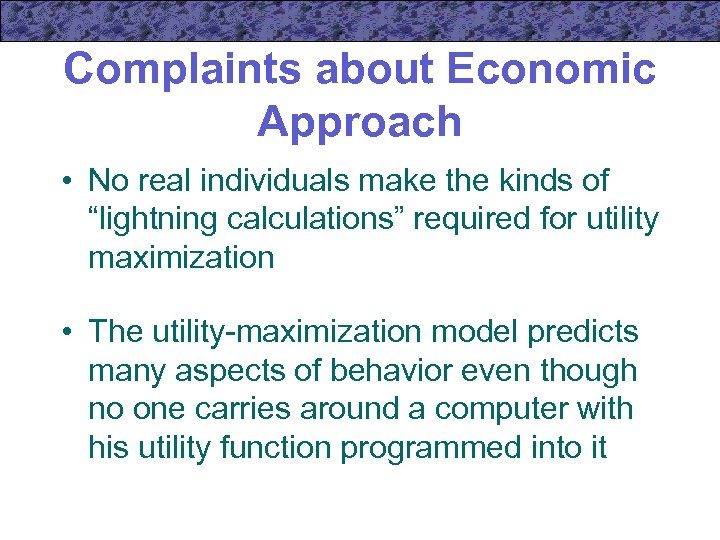Complaints about Economic Approach • No real individuals make the kinds of “lightning calculations” required for utility maximization • The utility-maximization model predicts many aspects of behavior even though no one carries around a computer with his utility function programmed into it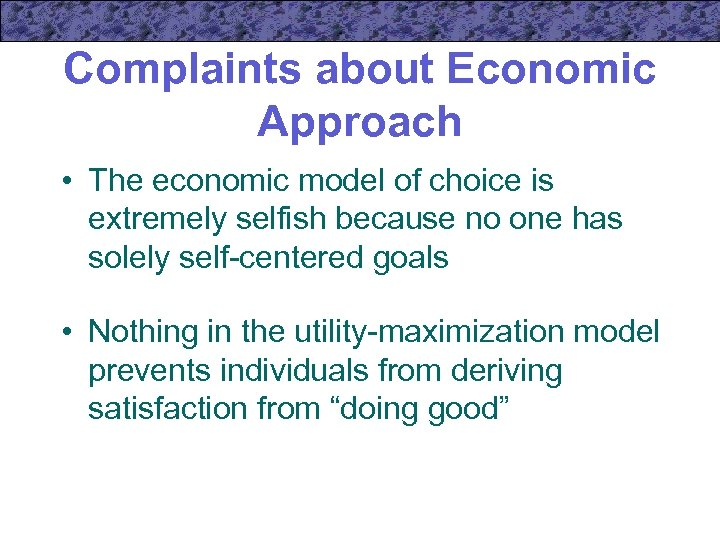Complaints about Economic Approach • The economic model of choice is extremely selfish because no one has solely self-centered goals • Nothing in the utility-maximization model prevents individuals from deriving satisfaction from “doing good”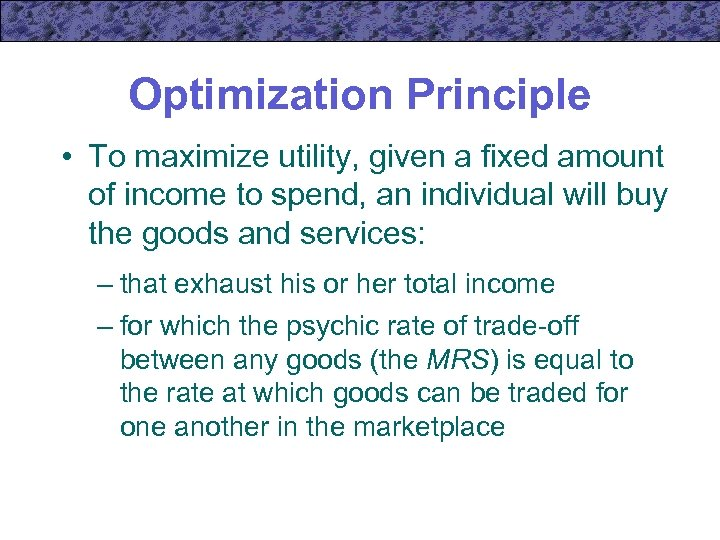Optimization Principle • To maximize utility, given a fixed amount of income to spend, an individual will buy the goods and services: – that exhaust his or her total income – for which the psychic rate of trade-off between any goods (the MRS) is equal to the rate at which goods can be traded for one another in the marketplace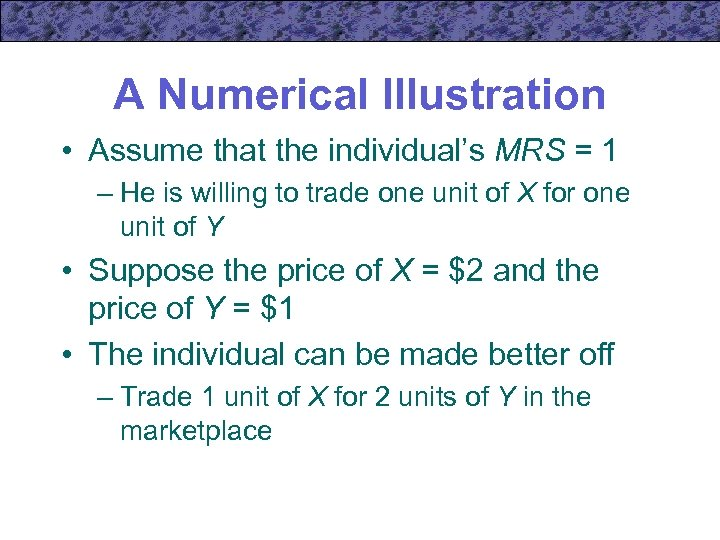A Numerical Illustration • Assume that the individual’s MRS = 1 – He is willing to trade one unit of X for one unit of Y • Suppose the price of X = \$2 and the price of Y = \$1 • The individual can be made better off – Trade 1 unit of X for 2 units of Y in the marketplace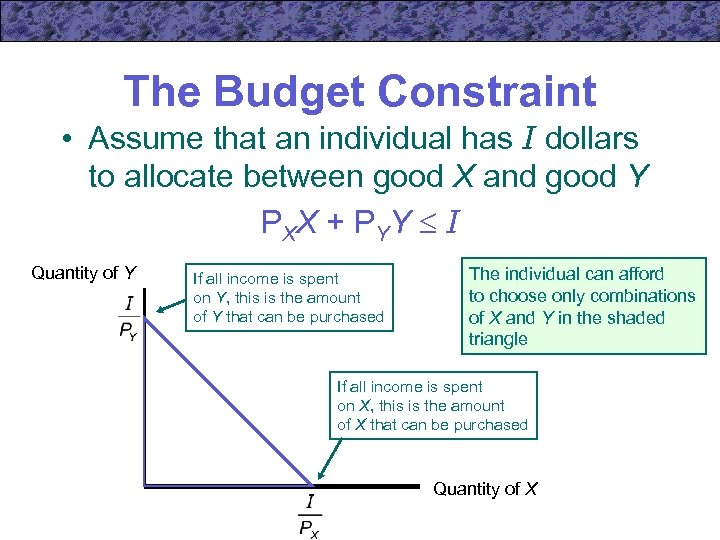The Budget Constraint • Assume that an individual has I dollars to allocate between good X and good Y P XX + P YY I Quantity of Y If all income is spent on Y, this is the amount of Y that can be purchased The individual can afford to choose only combinations of X and Y in the shaded triangle If all income is spent on X, this is the amount of X that can be purchased Quantity of X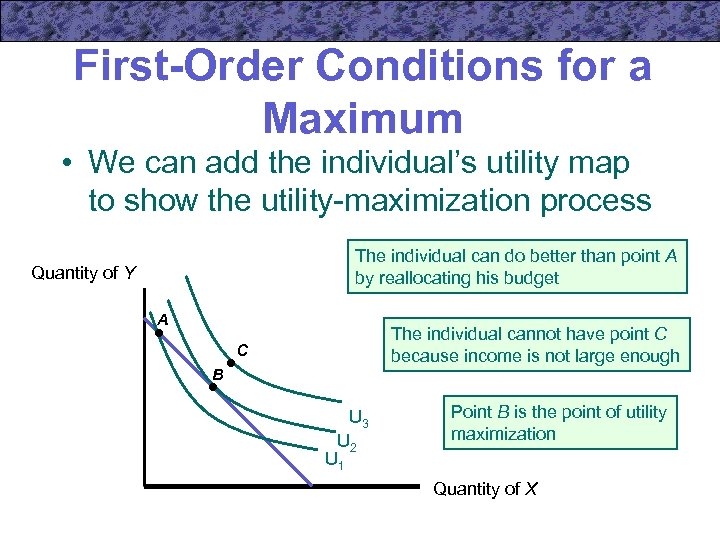First-Order Conditions for a Maximum • We can add the individual’s utility map to show the utility-maximization process The individual can do better than point A by reallocating his budget Quantity of Y A The individual cannot have point C because income is not large enough C B U 3 U 2 U 1 Point B is the point of utility maximization Quantity of X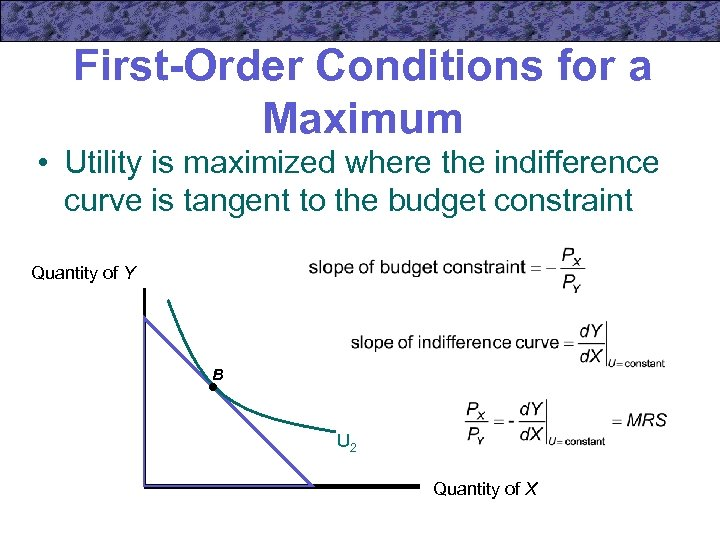First-Order Conditions for a Maximum • Utility is maximized where the indifference curve is tangent to the budget constraint Quantity of Y B U 2 Quantity of X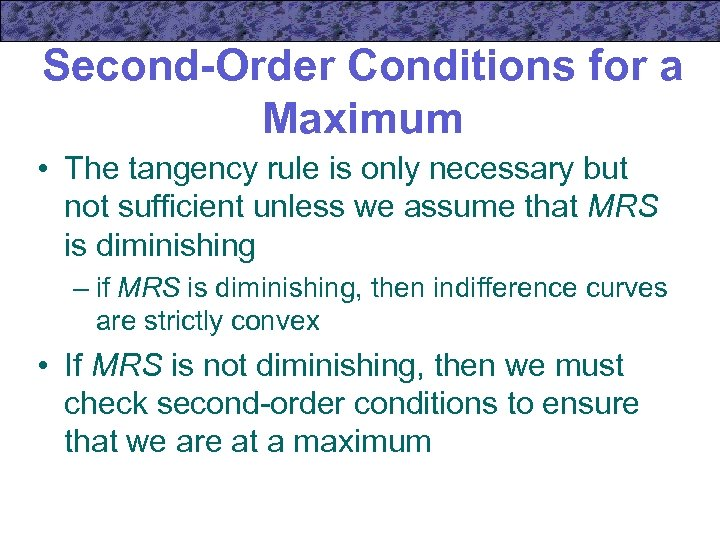Second-Order Conditions for a Maximum • The tangency rule is only necessary but not sufficient unless we assume that MRS is diminishing – if MRS is diminishing, then indifference curves are strictly convex • If MRS is not diminishing, then we must check second-order conditions to ensure that we are at a maximum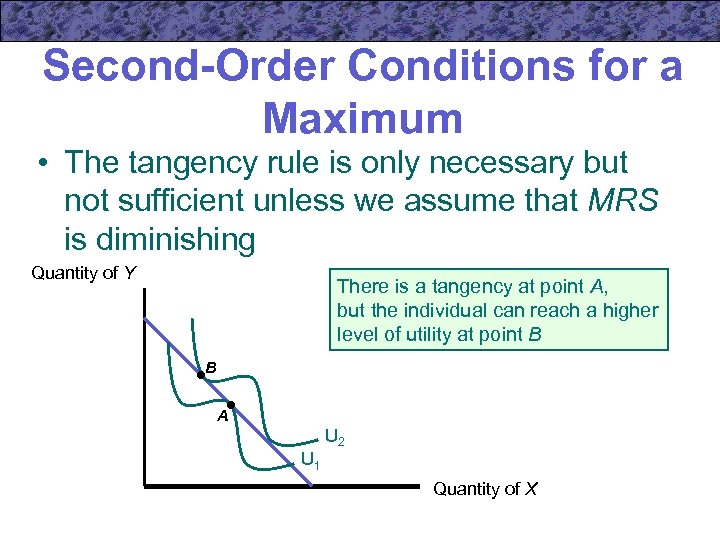Second-Order Conditions for a Maximum • The tangency rule is only necessary but not sufficient unless we assume that MRS is diminishing Quantity of Y There is a tangency at point A, but the individual can reach a higher level of utility at point B B A U 2 U 1 Quantity of X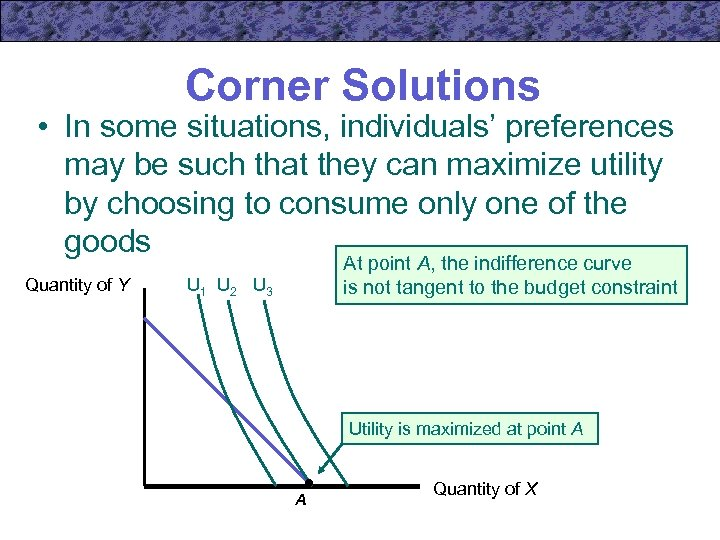Corner Solutions • In some situations, individuals’ preferences may be such that they can maximize utility by choosing to consume only one of the goods Quantity of Y At point A, the indifference curve is not tangent to the budget constraint U 1 U 2 U 3 Utility is maximized at point A A Quantity of X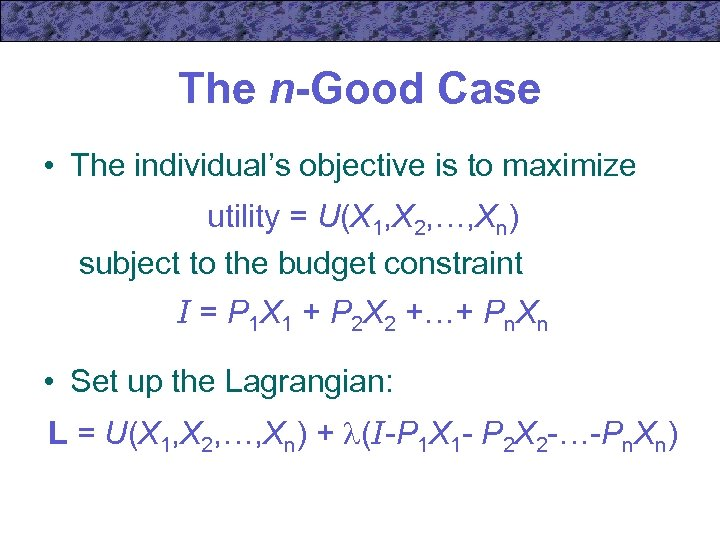The n-Good Case • The individual’s objective is to maximize utility = U(X 1, X 2, …, Xn) subject to the budget constraint I = P 1 X 1 + P 2 X 2 +…+ Pn. Xn • Set up the Lagrangian: L = U(X 1, X 2, …, Xn) + (I-P 1 X 1 - P 2 X 2 -…-Pn. Xn)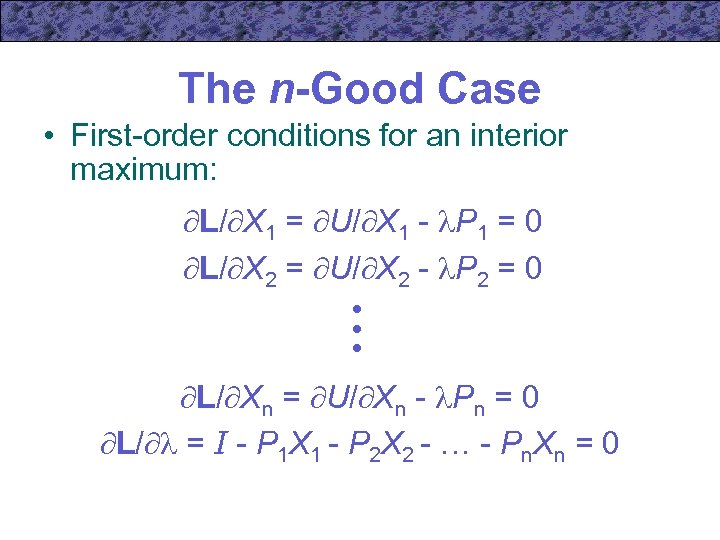The n-Good Case • First-order conditions for an interior maximum: L/ X 1 = U/ X 1 - P 1 = 0 L/ X 2 = U/ X 2 - P 2 = 0 • • • L/ Xn = U/ Xn - Pn = 0 L/ = I - P 1 X 1 - P 2 X 2 - … - Pn. Xn = 0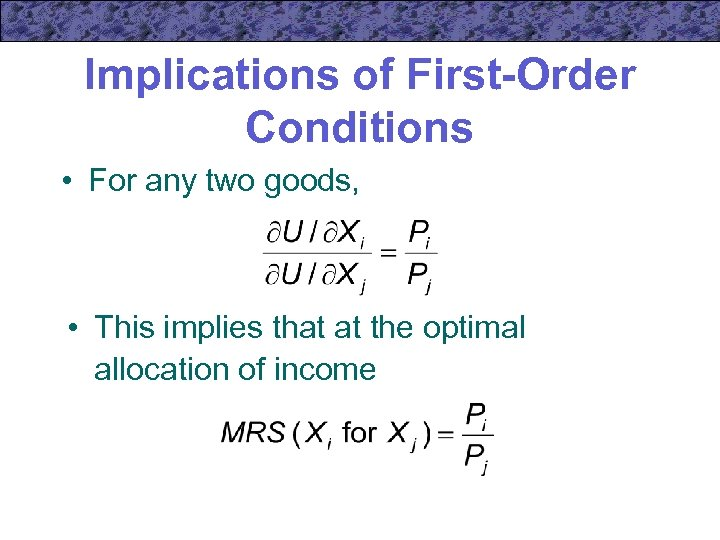Implications of First-Order Conditions • For any two goods, • This implies that at the optimal allocation of income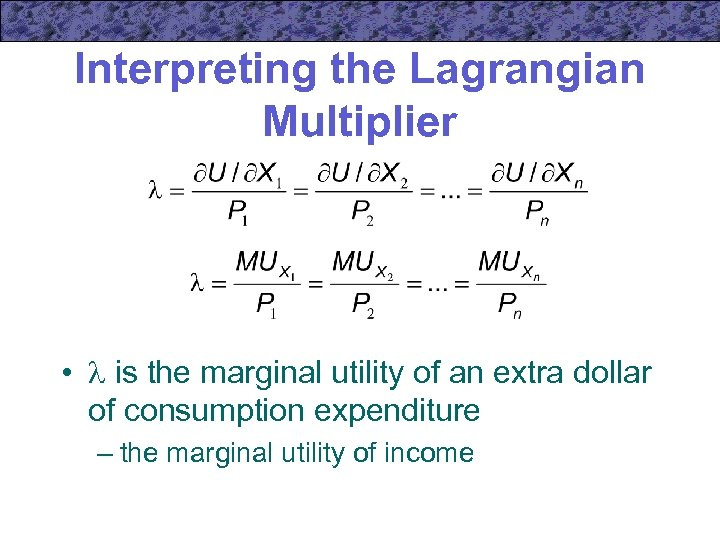Interpreting the Lagrangian Multiplier • is the marginal utility of an extra dollar of consumption expenditure – the marginal utility of income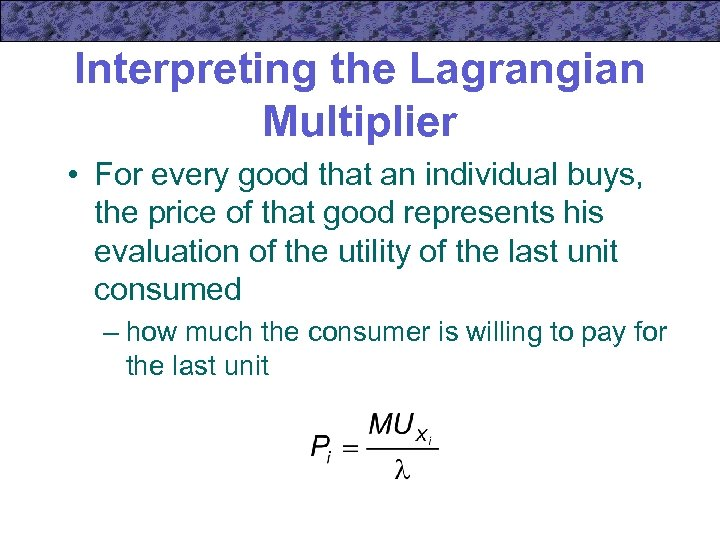Interpreting the Lagrangian Multiplier • For every good that an individual buys, the price of that good represents his evaluation of the utility of the last unit consumed – how much the consumer is willing to pay for the last unit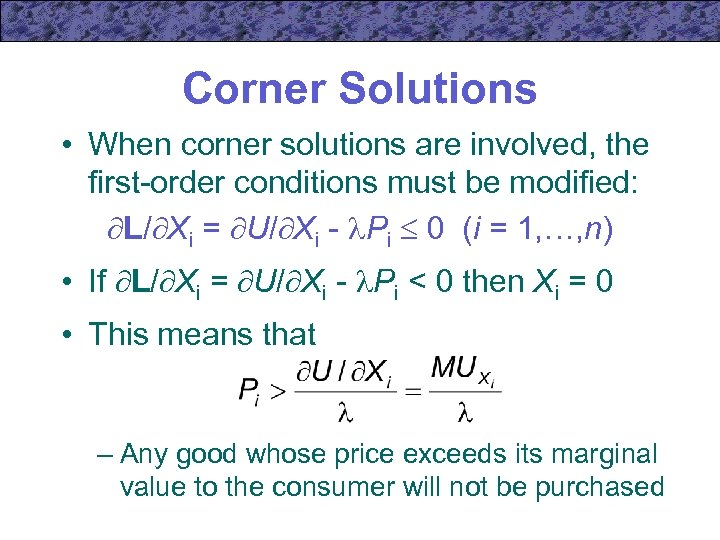Corner Solutions • When corner solutions are involved, the first-order conditions must be modified: L/ Xi = U/ Xi - Pi 0 (i = 1, …, n) • If L/ Xi = U/ Xi - Pi < 0 then Xi = 0 • This means that – Any good whose price exceeds its marginal value to the consumer will not be purchased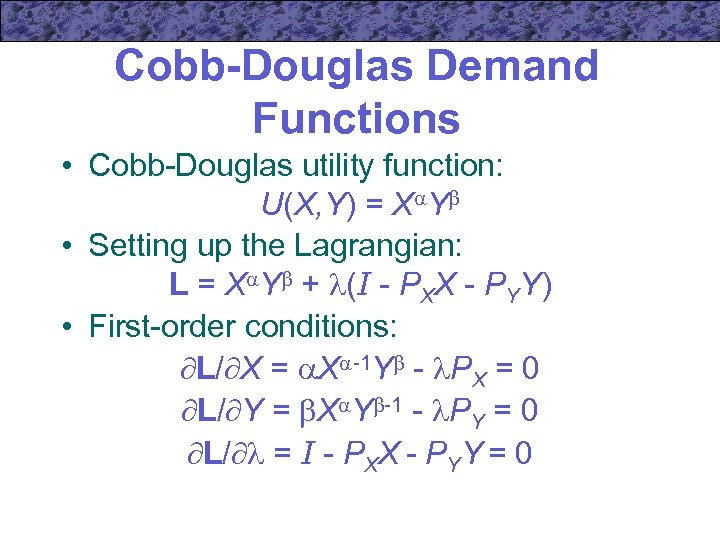Cobb-Douglas Demand Functions • Cobb-Douglas utility function: U(X, Y) = X Y • Setting up the Lagrangian: L = X Y + (I - PXX - PYY) • First-order conditions: L/ X = X -1 Y - PX = 0 L/ Y = X Y -1 - PY = 0 L/ = I - PXX - PYY = 0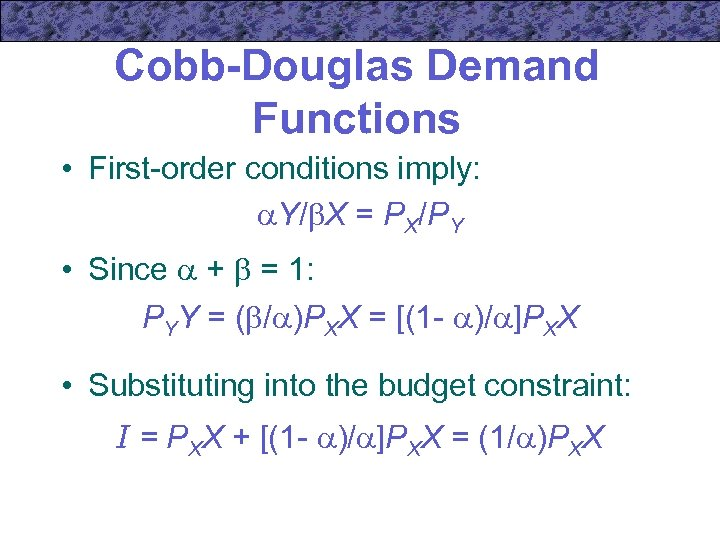Cobb-Douglas Demand Functions • First-order conditions imply: Y/ X = PX/PY • Since + = 1: PYY = ( / )PXX = [(1 - )/ ]PXX • Substituting into the budget constraint: I = PXX + [(1 - )/ ]PXX = (1/ )PXX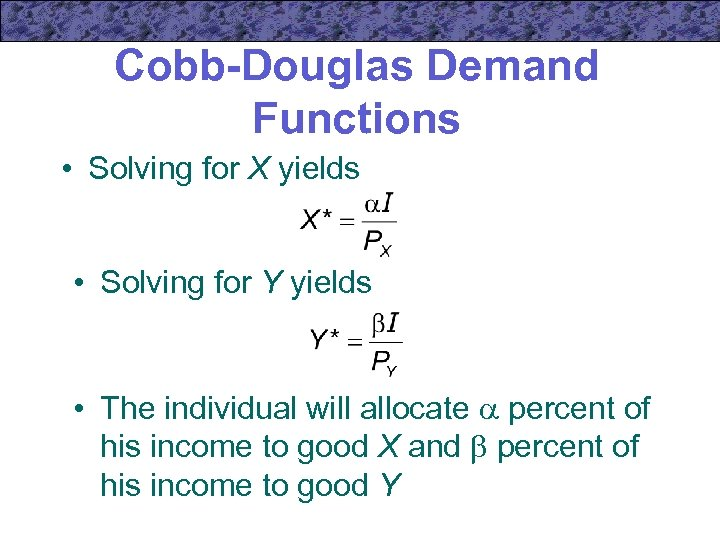Cobb-Douglas Demand Functions • Solving for X yields • Solving for Y yields • The individual will allocate percent of his income to good X and percent of his income to good Y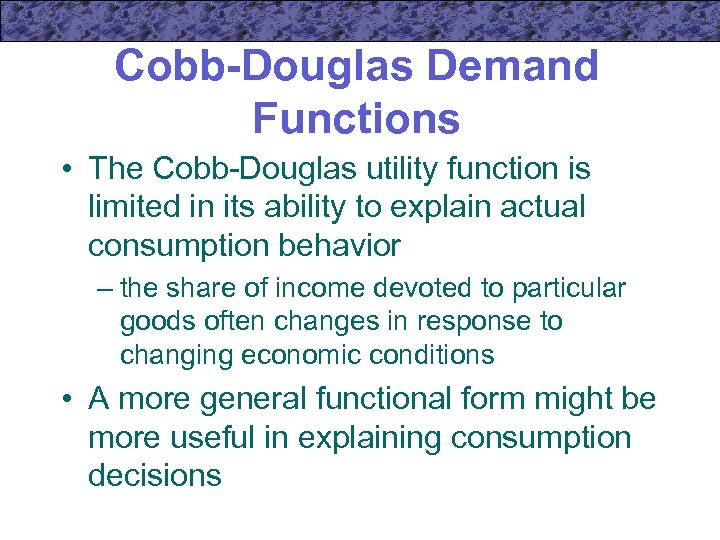Cobb-Douglas Demand Functions • The Cobb-Douglas utility function is limited in its ability to explain actual consumption behavior – the share of income devoted to particular goods often changes in response to changing economic conditions • A more general functional form might be more useful in explaining consumption decisions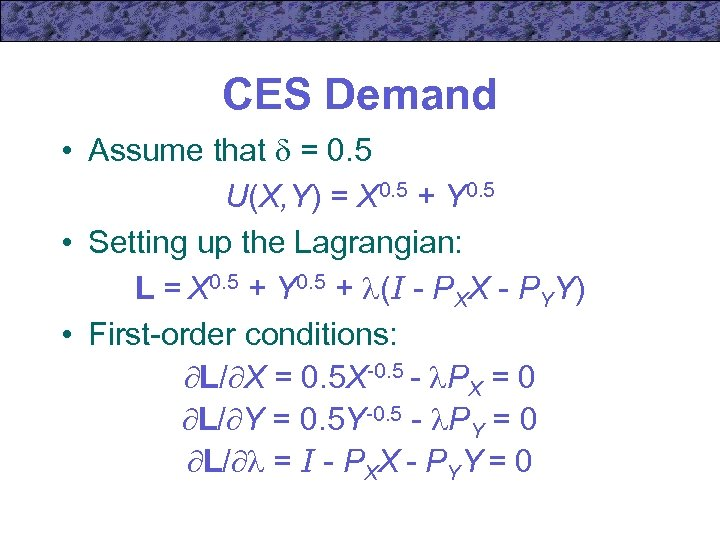CES Demand • Assume that = 0. 5 U(X, Y) = X 0. 5 + Y 0. 5 • Setting up the Lagrangian: L = X 0. 5 + Y 0. 5 + (I - PXX - PYY) • First-order conditions: L/ X = 0. 5 X-0. 5 - PX = 0 L/ Y = 0. 5 Y-0. 5 - PY = 0 L/ = I - PXX - PYY = 0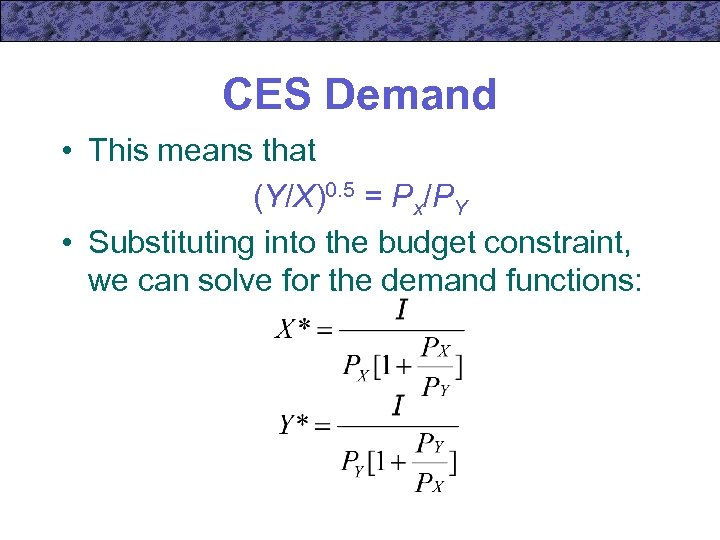CES Demand • This means that (Y/X)0. 5 = Px/PY • Substituting into the budget constraint, we can solve for the demand functions: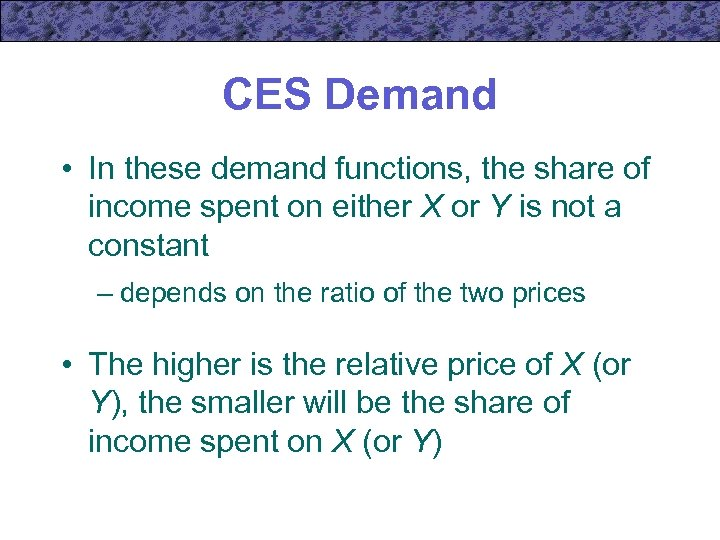CES Demand • In these demand functions, the share of income spent on either X or Y is not a constant – depends on the ratio of the two prices • The higher is the relative price of X (or Y), the smaller will be the share of income spent on X (or Y)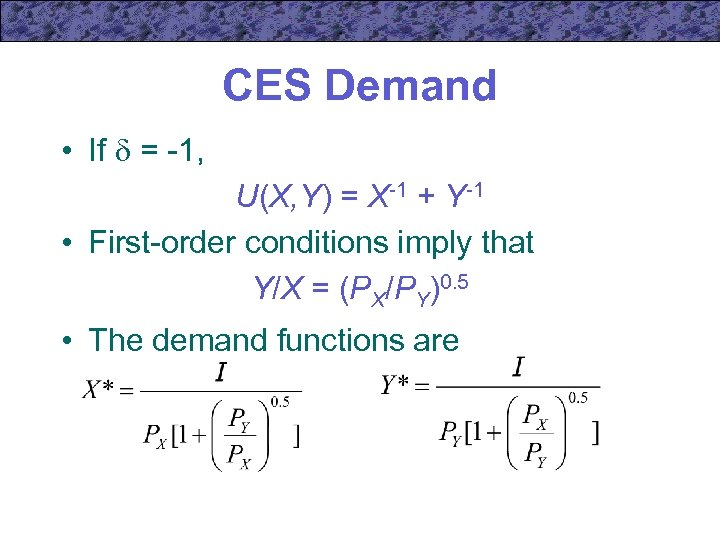CES Demand • If = -1, U(X, Y) = X-1 + Y-1 • First-order conditions imply that Y/X = (PX/PY)0. 5 • The demand functions are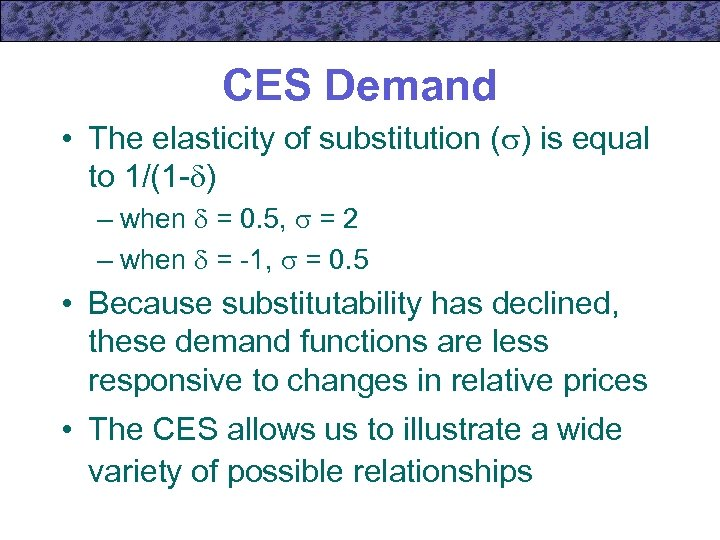CES Demand • The elasticity of substitution ( ) is equal to 1/(1 - ) – when = 0. 5, = 2 – when = -1, = 0. 5 • Because substitutability has declined, these demand functions are less responsive to changes in relative prices • The CES allows us to illustrate a wide variety of possible relationships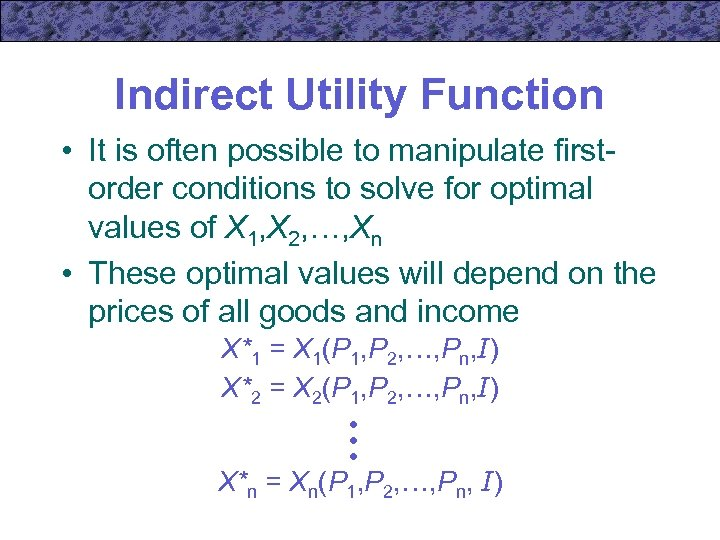Indirect Utility Function • It is often possible to manipulate firstorder conditions to solve for optimal values of X 1, X 2, …, Xn • These optimal values will depend on the prices of all goods and income X*1 = X 1(P 1, P 2, …, Pn, I) X*2 = X 2(P 1, P 2, …, Pn, I) • • • X*n = Xn(P 1, P 2, …, Pn, I)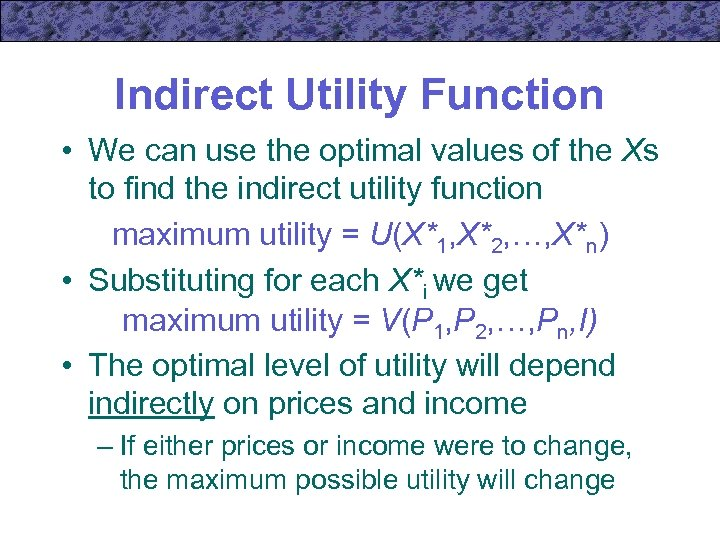Indirect Utility Function • We can use the optimal values of the Xs to find the indirect utility function maximum utility = U(X*1, X*2, …, X*n) • Substituting for each X*i we get maximum utility = V(P 1, P 2, …, Pn, I) • The optimal level of utility will depend indirectly on prices and income – If either prices or income were to change, the maximum possible utility will change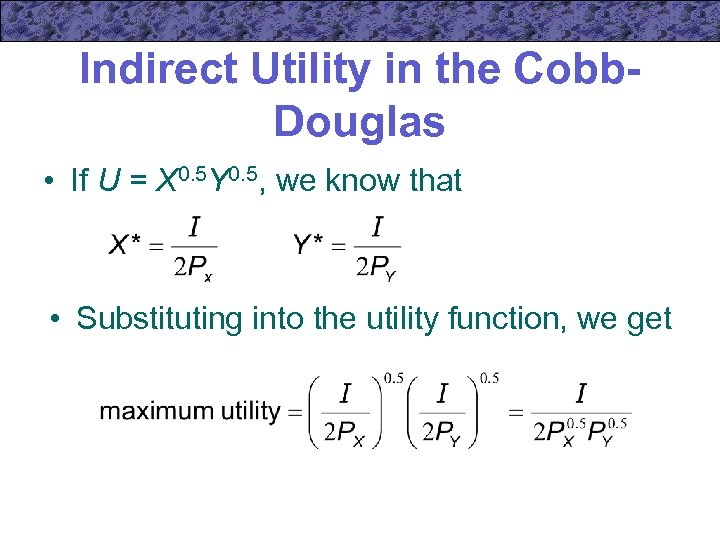Indirect Utility in the Cobb. Douglas • If U = X 0. 5 Y 0. 5, we know that • Substituting into the utility function, we get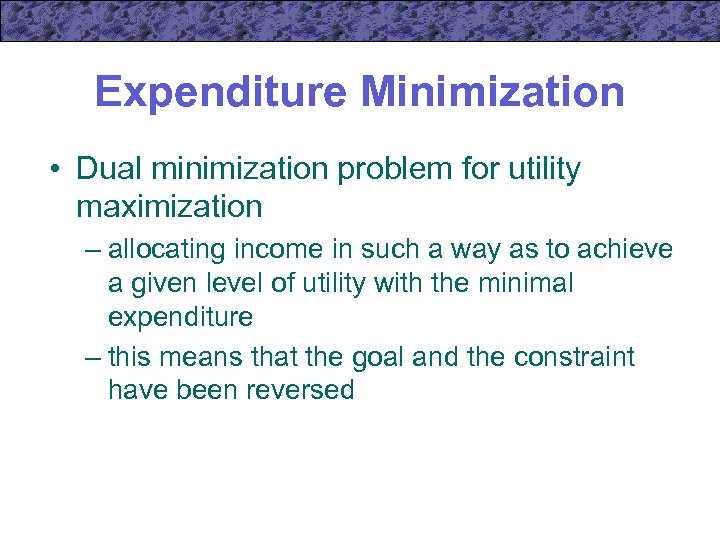Expenditure Minimization • Dual minimization problem for utility maximization – allocating income in such a way as to achieve a given level of utility with the minimal expenditure – this means that the goal and the constraint have been reversed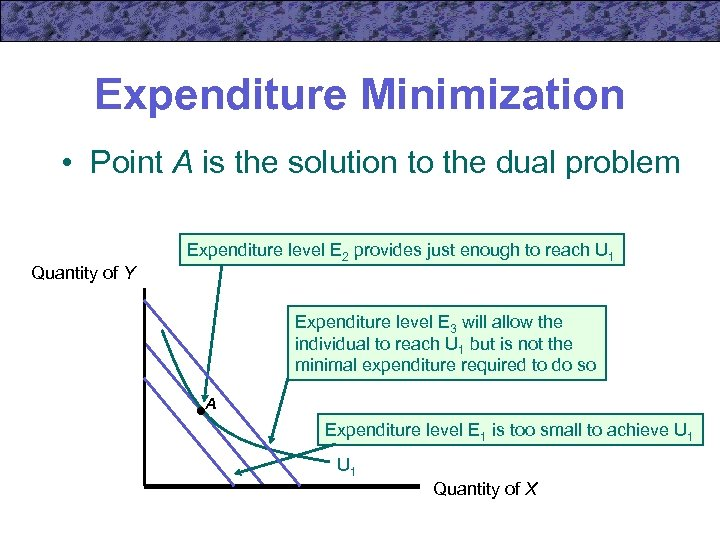Expenditure Minimization • Point A is the solution to the dual problem Quantity of Y Expenditure level E 2 provides just enough to reach U 1 Expenditure level E 3 will allow the individual to reach U 1 but is not the minimal expenditure required to do so A Expenditure level E 1 is too small to achieve U 1 Quantity of XExpenditure Minimization • The individual’s problem is to choose X 1, X 2, …, Xn to minimize E = P 1 X 1 + P 2 X 2 +…+Pn. Xn subject to the constraint U 1 = U(X 1, X 2, …, Un) • The optimal amounts of X 1, X 2, …, Xn will depend on the prices of the goods and the required utility level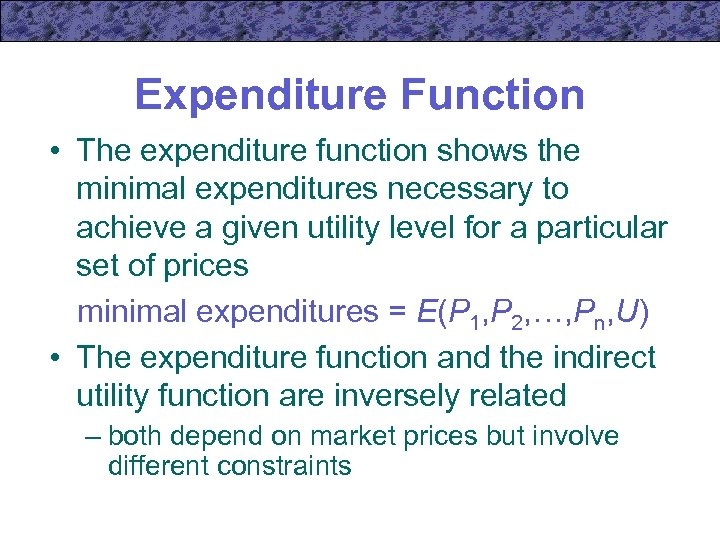Expenditure Function • The expenditure function shows the minimal expenditures necessary to achieve a given utility level for a particular set of prices minimal expenditures = E(P 1, P 2, …, Pn, U) • The expenditure function and the indirect utility function are inversely related – both depend on market prices but involve different constraints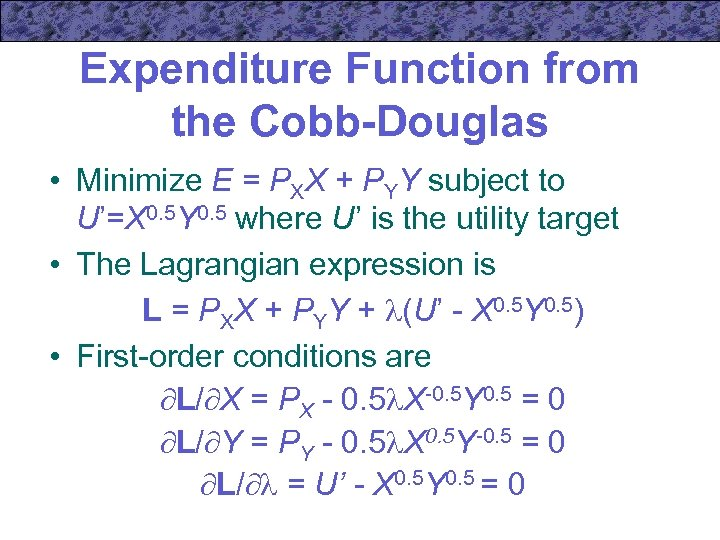Expenditure Function from the Cobb-Douglas • Minimize E = PXX + PYY subject to U’=X 0. 5 Y 0. 5 where U’ is the utility target • The Lagrangian expression is L = PXX + PYY + (U’ - X 0. 5 Y 0. 5) • First-order conditions are L/ X = PX - 0. 5 X-0. 5 Y 0. 5 = 0 L/ Y = PY - 0. 5 X 0. 5 Y-0. 5 = 0 L/ = U’ - X 0. 5 Y 0. 5 = 0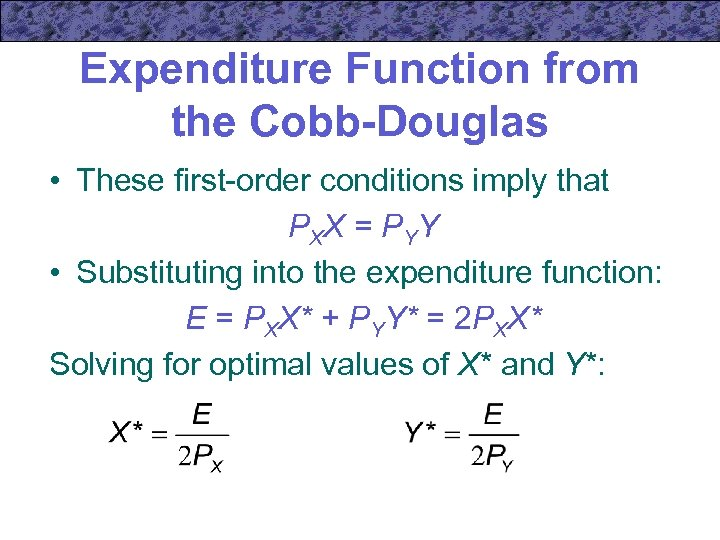Expenditure Function from the Cobb-Douglas • These first-order conditions imply that P XX = P YY • Substituting into the expenditure function: E = PXX* + PYY* = 2 PXX* Solving for optimal values of X* and Y*: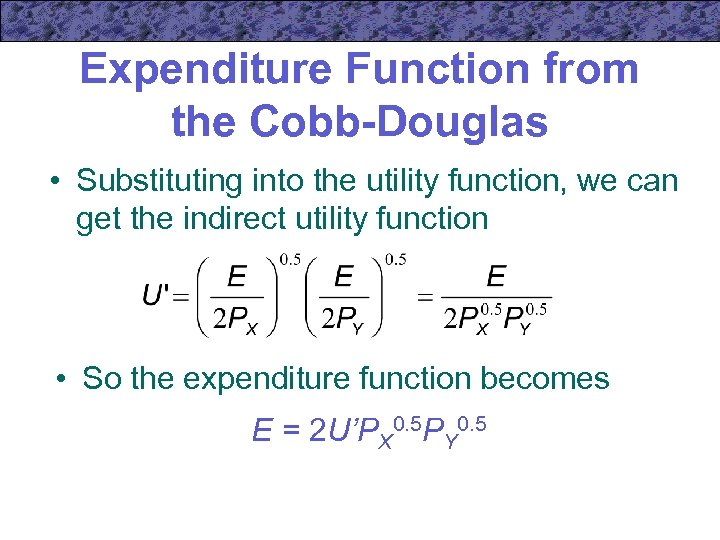Expenditure Function from the Cobb-Douglas • Substituting into the utility function, we can get the indirect utility function • So the expenditure function becomes E = 2 U’PX 0. 5 PY 0. 5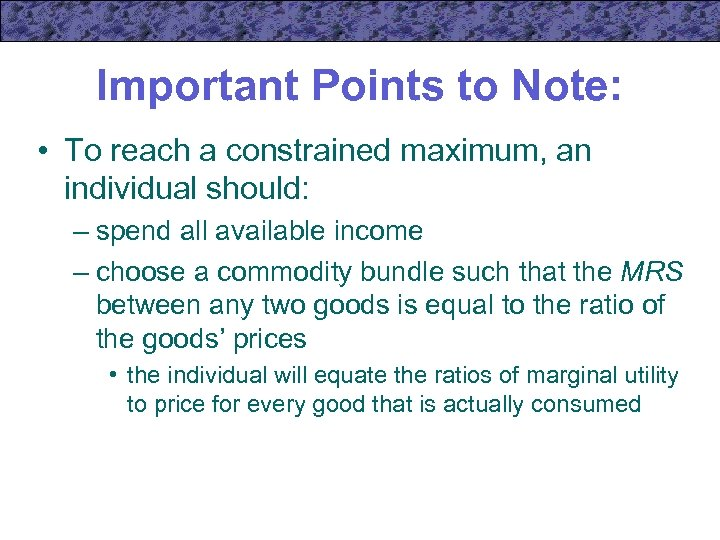Important Points to Note: • To reach a constrained maximum, an individual should: – spend all available income – choose a commodity bundle such that the MRS between any two goods is equal to the ratio of the goods’ prices • the individual will equate the ratios of marginal utility to price for every good that is actually consumed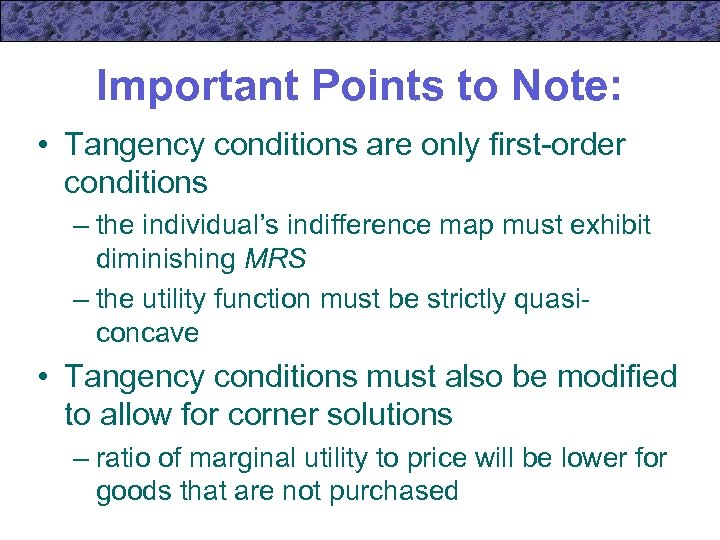Important Points to Note: • Tangency conditions are only first-order conditions – the individual’s indifference map must exhibit diminishing MRS – the utility function must be strictly quasiconcave • Tangency conditions must also be modified to allow for corner solutions – ratio of marginal utility to price will be lower for goods that are not purchased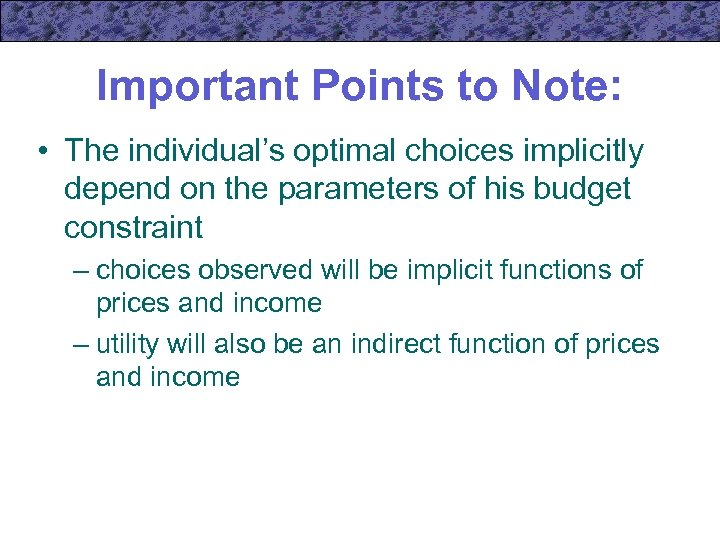Important Points to Note: • The individual’s optimal choices implicitly depend on the parameters of his budget constraint – choices observed will be implicit functions of prices and income – utility will also be an indirect function of prices and income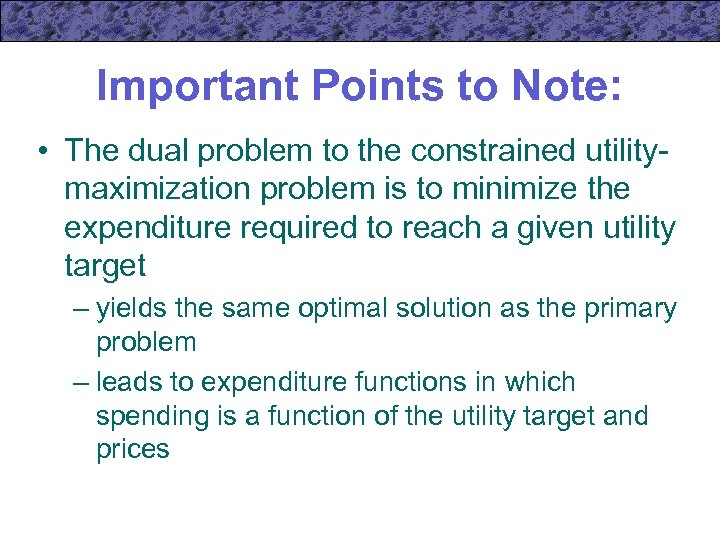Important Points to Note: • The dual problem to the constrained utilitymaximization problem is to minimize the expenditure required to reach a given utility target – yields the same optimal solution as the primary problem – leads to expenditure functions in which spending is a function of the utility target and prices